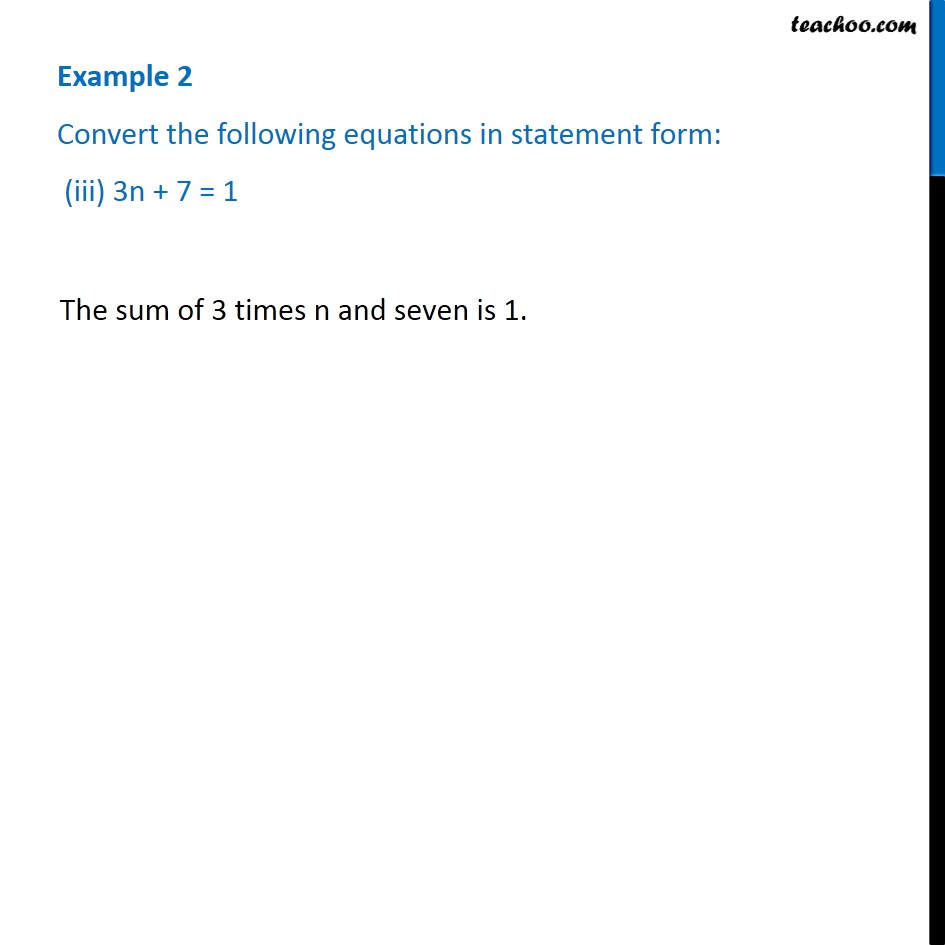1. Chapter 4 Class 7 Simple Equations
2. Serial order wise
3. Examples

Transcript

Example 2 Convert the following equations in statement form: (iii) 3n + 7 = 1 The sum of 3 times n and seven is 1.